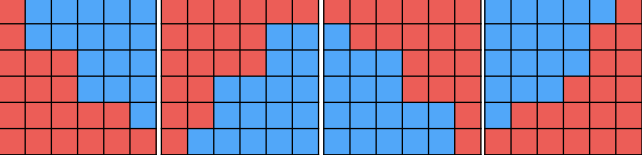# 【2018.9.20】JOI 2017 Final T3「JOIOI 王国 / The Kingdom of JOIOI」

### 题目描述

JOIOI 王国是一个 $H$ 行 $W$ 列的长方形网格，每个 $1\times 1$ 的子网格都是一个正方形的小区块。为了提高管理效率，我们决定把整个国家划分成两个省 $JOI$ 和 $IOI$ 。

• 区块不能被分割为两半，一半属 $JOI$ 省，一半属 $IOI$ 省。
• 每个省必须包含至少一个区块，每个区块也必须属于且只属于其中一个省。
• 同省的任意两个小区块互相连接。
• 对于每一行/列，如果我们将这一行/列单独取出，这一行/列里同省的任意两个区块互相连接。这一行/列内的所有区块可以全部属于一个省。

### 样例

#### 样例输入 1

4 4
1 12 6 11
11 10 2 14
10 1 9 20
4 17 19 10

#### 样例输出 1

11

#### 样例解释 1

 $J$ $J$ $J$ $I$ $J$ $J$ $J$ $I$ $J$ $J$ $I$ $I$ $J$ $I$ $I$

 $J$ $J$ $I$ $I$ $J$ $J$ $J$ $I$ $J$ $J$ $I$ $I$ $J$ $I$ $I$ $I$

#### 样例输入 2

8 6
23 23 10 11 16 21
15 26 19 28 19 20
25 26 28 16 15 11
11 8 19 11 15 24
14 19 15 14 24 11
10 8 11 7 6 14
23 5 19 23 17 17
18 11 21 14 20 16

#### 样例输出 2

18

### 数据范围与提示• 同省的任意两个小区块互相连接。
• 对于每一行/列，如果我们将这一行/列单独取出，这一行/列里同省的任意两个区块互相连接。这一行/列内的所有区块可以全部属于一个省1 #include <cstdio>
2 #include <algorithm>
3 using namespace std;
4
5 int H, W, A;
6 int gmin, gmax;
7
8 bool check(int th)
9 {
10     int sep = 0;
11     for (int i = 0; i < H; ++i) {
12         for (int j = 0; j < W; ++j) {
13             if (A[i][j] < gmax - th) {
14                 sep = max(sep, j + 1);
15             }
16         }
17         for (int j = 0; j < W; ++j) {
18             if (gmin + th < A[i][j]) {
19                 if (j < sep) return false;
20             }
21         }
22     }
23     return true;
24 }
25 void flip_row()
26 {
27     for (int i = 0; i < H / 2; ++i) {
28         for (int j = 0; j < W; ++j) {
29             swap(A[i][j], A[H - 1 - i][j]);
30         }
31     }
32 }
33 void flip_col()
34 {
35     for (int i = 0; i < H; ++i) {
36         for (int j = 0; j < W / 2; ++j) {
37             swap(A[i][j], A[i][W - 1 - j]);
38         }
39     }
40 }
41 int solve()
42 {
43     int lo = 0, hi = gmax - gmin;
44     while (lo < hi) {
45         int mid = (lo + hi) / 2;
46         if (check(mid)) {
47             hi = mid;
48         } else {
49             lo = mid + 1;
50         }
51     }
52     return lo;
53 }
54 int main()
55 {
56     scanf("%d%d", &H, &W);
57     for (int i = 0; i < H; ++i) {
58         for (int j = 0; j < W; ++j) {
59             scanf("%d", &(A[i][j]));
60         }
61     }
62
63     gmin = gmax = A;
64     for (int i = 0; i < H; ++i) {
65         for (int j = 0; j < W; ++j) {
66             gmin = min(gmin, A[i][j]);
67             gmax = max(gmax, A[i][j]);
68         }
69     }
70
71         //翻转三次，得到四种情况
72     int ret = solve();
73     flip_row();
74     ret = min(ret, solve());
75     flip_col();
76     ret = min(ret, solve());
77     flip_row();
78     ret = min(ret, solve());
79     printf("%d\n", ret);
80     return 0;
81 }
View Code
posted @ 2018-09-21 17:10  大本营  阅读(546)  评论(0编辑  收藏  举报